# shapes¶

Submodule to generate basic geometric shapes.

## Arc¶

class vedo.shapes.Arc(center, point1, point2=None, normal=None, angle=None, invert=False, c='grey', alpha=1, res=48)[source]

Bases: vedo.mesh.Mesh

Build a 2D circular arc between points point1 and point2. If normal is specified then center is ignored, and normal vector, a starting point1 (polar vector) and an angle defining the arc length need to be assigned.

Arc spans the shortest angular sector point1 and point2, if invert=True, then the opposite happens.

length()[source]

Calculate length of the arc.

## Arrow¶

class vedo.shapes.Arrow(startPoint, endPoint, s=None, c='r', alpha=1, res=12)[source]

Bases: vedo.mesh.Mesh

Build a 3D arrow from startPoint to endPoint of section size s, expressed as the fraction of the window size.

Note

If s=None the arrow is scaled proportionally to its length## Arrow2D¶

class vedo.shapes.Arrow2D(startPoint, endPoint, shaftLength=0.8, shaftWidth=0.05, headLength=0.25, headWidth=0.2, fill=True, c='r', alpha=1)[source]

Bases: vedo.mesh.Mesh

Build a 2D arrow from startPoint to endPoint.

Parameters
• shaftLength (float) – fractional shaft length

• shaftWidth (float) – fractional shaft width

• fill (bool) – if False only generate the outline

## Arrows¶

vedo.shapes.Arrows(startPoints, endPoints=None, s=None, scale=1, c=None, alpha=1, res=12)[source]

Build arrows between two lists of points startPoints and endPoints. startPoints can be also passed in the form [[point1, point2], ...].

Color can be specified as a colormap which maps the size of the arrows.

Parameters
• s (float) – fix aspect-ratio of the arrow and scale its cross section

• scale (float) – apply a rescaling factor to the length

• c – color or color map name.

• alpha (float) – set transparency

• res (int) – set arrow resolution

## Arrows2D¶

vedo.shapes.Arrows2D(startPoints, endPoints=None, shaftLength=0.8, shaftWidth=0.09, headLength=None, headWidth=0.2, fill=True, scale=1, c=None, cmap=None, alpha=1)[source]

Build 2D arrows between two lists of points startPoints and endPoints. startPoints can be also passed in the form [[point1, point2], ...].

Color can be specified as a colormap which maps the size of the arrows.

Parameters
• shaftLength (float) – fractional shaft length

• shaftWidth (float) – fractional shaft width

• fill (bool) – if False only generate the outline

• scale (float) – apply a rescaling factor to the length

• c – color

• alpha (float) – set transparency

Example
from vedo import Grid, Arrows2D
g1 = Grid(sx=1, sy=1)
g2 = Grid(sx=1.2, sy=1.2).rotateZ(4)
arrs2d = Arrows2D(g1, g2, c='jet')
arrs2d.show(axes=1, bg='white')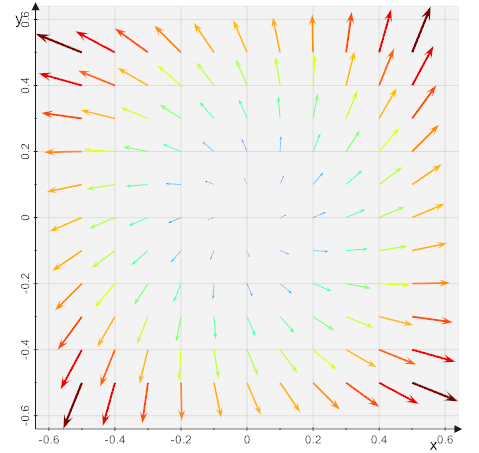## Bezier¶

vedo.shapes.Bezier(points, res=None)[source]

Generate the Bezier line that links the first to the last point.

Example
from vedo import *
import numpy as np
pts = np.random.randn(25,3)
for i,p in enumerate(pts):
p += [5*i, 15*sin(i/2), i*i*i/200]
show(Points(pts), Bezier(pts), axes=1)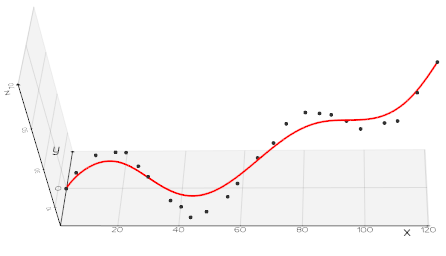## Box¶

class vedo.shapes.Box(pos=0, 0, 0, length=1, width=2, height=3, size=(), c='g', alpha=1)[source]

Bases: vedo.mesh.Mesh

Build a box of dimensions x=length, y=width and z=height. Alternatively dimensions can be defined by setting size keyword with a tuple.

## Circle¶

class vedo.shapes.Circle(pos=0, 0, 0, r=1, c='grey', alpha=1, res=120)[source]

Build a Circle of radius r.

## Cone¶

class vedo.shapes.Cone(pos=0, 0, 0, r=1, height=3, axis=0, 0, 1, c='dg', alpha=1, res=48)[source]

Bases: vedo.mesh.Mesh

Build a cone of specified radius r and height, centered at pos.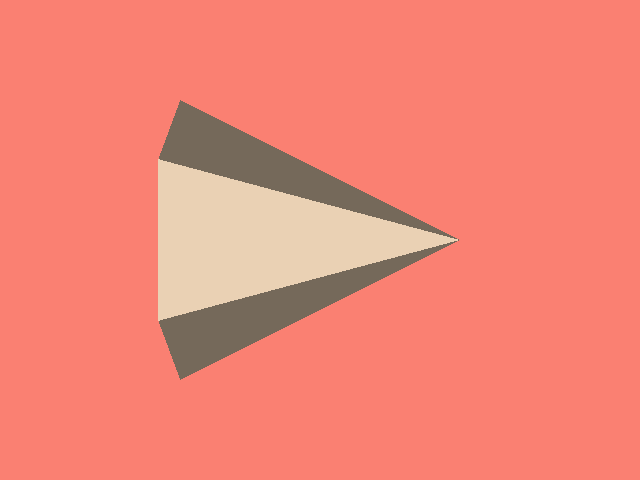## ConvexHull¶

vedo.shapes.ConvexHull(pts)[source]

Create the 2D/3D convex hull of a set of input points or input Mesh.

## Cross3D¶

vedo.shapes.Cross3D(pos=0, 0, 0, s=1.0, thickness=0.3, c='b', alpha=1)[source]

Build a 3D cross shape, mainly useful as a 3D marker.

## Cube¶

vedo.shapes.Cube(pos=0, 0, 0, side=1, c='g', alpha=1)[source]

Build a cube of size side.

## CubicGrid¶

vedo.shapes.CubicGrid(pos=0, 0, 0, n=10, 10, 10, spacing=(), c='lightgrey', alpha=0.1)[source]

Build a cubic Mesh made o n small quads in the 3 axis directions.

Parameters
• pos (list) – position of the left bottom corner

• n (int) – number of subdivisions

• spacing (list) – size of the side of the single quad in the 3 directions

## Cylinder¶

class vedo.shapes.Cylinder(pos=0, 0, 0, r=1, height=2, axis=0, 0, 1, c='teal', alpha=1, res=24)[source]

Bases: vedo.mesh.Mesh

Build a cylinder of specified height and radius r, centered at pos.

If pos is a list of 2 Points, e.g. pos=[v1,v2], build a cylinder with base centered at v1 and top at v2.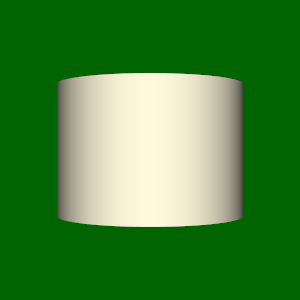## DashedLine¶

class vedo.shapes.DashedLine(p0, p1=None, spacing=0.1, closed=False, c='red', alpha=1, lw=2)[source]

Build a dashed line segment between points p0 and p1. If p0 is a list of points returns the line connecting them. A 2D set of coords can also be passed as p0=[x..], p1=[y..].

Parameters
• closed (bool) – join last to first point

• spacing (float) – relative size of the dash.

• c (int, str, list) – color name, number, or list of [R,G,B] colors.

• alpha (float) – transparency in range [0,1].

• lw – line width.

## Disc¶

class vedo.shapes.Disc(pos=0, 0, 0, r1=0.5, r2=1, c='coral', alpha=1, res=12)[source]

Bases: vedo.mesh.Mesh

Build a 2D disc of inner radius r1 and outer radius r2.

Parameters

res (list) – resolution in R and Phi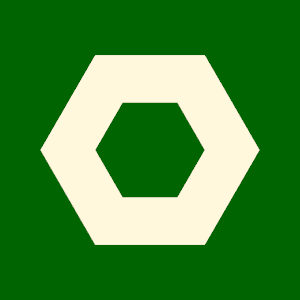## Earth¶

class vedo.shapes.Earth(style=1, r=1)[source]

Bases: vedo.mesh.Mesh

Build a textured mesh representing the Earth.

## Ellipsoid¶

class vedo.shapes.Ellipsoid(pos=0, 0, 0, axis1=1, 0, 0, axis2=0, 2, 0, axis3=0, 0, 3, c='c', alpha=1, res=24)[source]

Bases: vedo.mesh.Mesh

Build a 3D ellipsoid centered at position pos.

Note

axis1 and axis2 are only used to define sizes and one azimuth angle.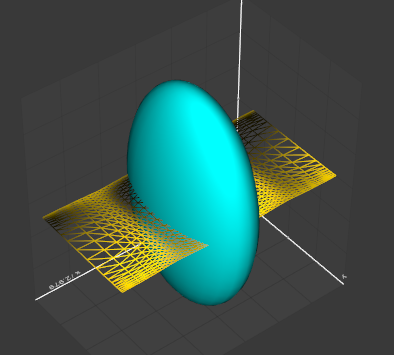asphericity()[source]

Return a measure of how different an ellipsoid is froma sphere. Values close to zero correspond to a spheric object.

asphericity_error()[source]

Calculate statistical error on the asphericity value.

Errors on the main axes are stored in Ellipsoid.va_error Ellipsoid.vb_error Ellipsoid.vc_error

## FlatArrow¶

vedo.shapes.FlatArrow(line1, line2, c='m', alpha=1, tipSize=1, tipWidth=1)[source]

Build a 2D arrow in 3D space by joining two close lines.

## Glyph¶

class vedo.shapes.Glyph(mesh, glyphObj, orientationArray=None, scaleByScalar=False, scaleByVectorSize=False, scaleByVectorComponents=False, colorByScalar=False, colorByVectorSize=False, tol=0, c='white', alpha=1)[source]

Bases: vedo.mesh.Mesh

At each vertex of a mesh, another mesh - a ‘glyph’ - is shown with various orientation options and coloring. The input mesh can also be a simple list of 2D or 3D coordinates. Color can be specified as a colormap which maps the size of the orientation vectors in orientationArray.

Parameters
• orientationArray (list, str, vtkAbstractArray) – list of vectors, vtkAbstractArray or the name of an already existing points array.

• scaleByScalar (bool) – glyph mesh is scaled by the active scalars.

• scaleByVectorSize (bool) – glyph mesh is scaled by the size of the vectors.

• scaleByVectorComponents (bool) – glyph mesh is scaled by the 3 vectors components.

• colorByScalar (bool) – glyph mesh is colored based on the scalar value.

• colorByVectorSize (bool) – glyph mesh is colored based on the vector size.

• tol (float) – set a minimum separation between two close glyphs (not compatible with orientationArray being a list).

## Grid¶

class vedo.shapes.Grid(pos=0, 0, 0, normal=0, 0, 1, sx=1, sy=1, sz=0, c='gray', alpha=1, lw=1, resx=10, resy=10)[source]

Bases: vedo.mesh.Mesh

Return an even or uneven 2D grid at z=0.

Parameters
• sx (float,list) – if a float is provided it is interpreted as the total size along x, if a list of coords is provided they are interpreted as the vertices of the grid along x. In this case keyword resx is ignored (see example below).

• sy (float,list) – see above.

• lw (float) – line width.

• resx (int) – resolution along x, e.i. the number of axis subdivisions.

Example
from vedo import *
xcoords = arange(0, 2, 0.2)
ycoords = arange(0, 1, 0.2)
sqrtx = sqrt(xcoords)
grid = Grid(sx=sqrtx, sy=ycoords)
grid.show(axes=8)


## Hyperboloid¶

class vedo.shapes.Hyperboloid(pos=0, 0, 0, a2=1, value=0.5, height=1, c='m', alpha=1, res=100)[source]

Bases: vedo.mesh.Mesh

Build a hyperboloid of specified aperture a2 and height, centered at pos.

Full volumetric expression is:

$$F(x,y,z)=a_0x^2+a_1y^2+a_2z^2+a_3xy+a_4yz+a_5xz+ a_6x+a_7y+a_8z+a_9$$

## KSpline¶

class vedo.shapes.KSpline(points, continuity=0, tension=0, bias=0, closed=False, res=None)[source]

Return a Kochanek spline which runs exactly through all the input points.

Parameters
• continuity (float) – changes the sharpness in change between tangents

• tension (float) – changes the length of the tangent vector

• bias (float) – changes the direction of the tangent vector

• closed (bool) – join last to first point to produce a closed curve

• res (int) – resolution of the output line. Default is 20 times the number of input points.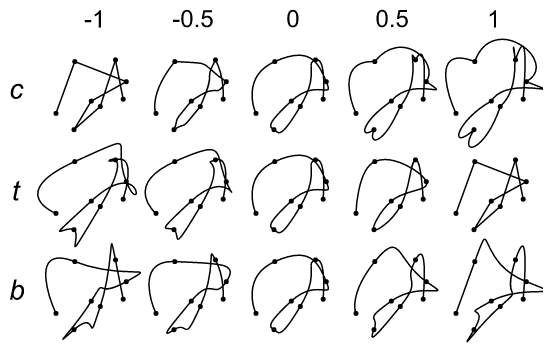## Latex¶

class vedo.shapes.Latex(formula, pos=0, 0, 0, c='k', s=1, bg=None, alpha=1, res=30, usetex=False, fromweb=False)[source]

Render Latex formulas.

Parameters
• formula (str) – latex text string

• pos (list) – position coordinates in space

• c – face color

• bg – background color box

• res (int) – dpi resolution

• usetex (bool) – use latex compiler of matplotlib

• fromweb – retrieve the latex image from online server (codecogs)

You can access the latex formula in Latex.formula’.

## Line¶

class vedo.shapes.Line(p0, p1=None, closed=False, c='r', alpha=1, lw=1, res=None)[source]

Bases: vedo.mesh.Mesh

Build the line segment between points p0 and p1. If p0 is a list of points returns the line connecting them. A 2D set of coords can also be passed as p0=[x..], p1=[y..].

Parameters
• closed (bool) – join last to first point

• c (int, str, list) – color name, number, or list of [R,G,B] colors.

• alpha (float) – transparency in range [0,1].

• lw – line width.

• res (int) – number of intermediate points in the segment

length()[source]

Calculate length of line.

## Lines¶

class vedo.shapes.Lines(startPoints, endPoints=None, c='gray', alpha=1, lw=1, dotted=False, scale=1)[source]

Build the line segments between two lists of points startPoints and endPoints. startPoints can be also passed in the form [[point1, point2], ...].

Parameters

scale (float) – apply a rescaling factor to the lengths.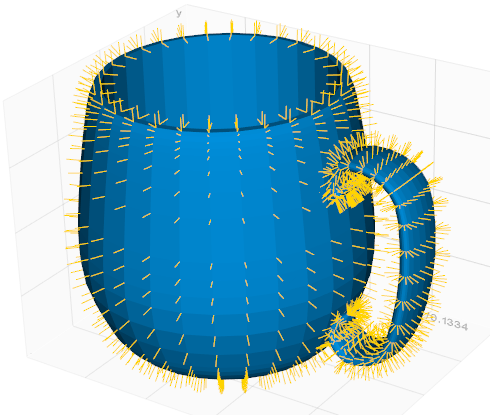## Marker¶

vedo.shapes.Marker(symbol, pos=0, 0, 0, c='lb', alpha=1, s=0.1, filled=True)[source]

Generate a marker shape. Can be used in association with Glyph.

## NormalLines¶

vedo.shapes.NormalLines(mesh, ratio=1, atCells=True, scale=1)[source]

Build an Mesh made of the normals at cells shown as lines.

if atCells is False normals are shown at vertices.

## Paraboloid¶

class vedo.shapes.Paraboloid(pos=0, 0, 0, r=1, height=1, c='cyan', alpha=1, res=50)[source]

Bases: vedo.mesh.Mesh

Build a paraboloid of specified height and radius r, centered at pos.

Note

Full volumetric expression is:

$$F(x,y,z)=a_0x^2+a_1y^2+a_2z^2+a_3xy+a_4yz+a_5xz+ a_6x+a_7y+a_8z+a_9$$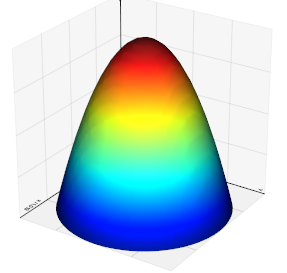## ParametricShape¶

class vedo.shapes.ParametricShape(name, c='powderblue', alpha=1, res=51)[source]

Bases: vedo.mesh.Mesh

A set of built-in shapes mainly for illustration purposes.

Name can be an integer or a string in this list:

[‘Boy’, ‘ConicSpiral’, ‘CrossCap’, ‘Dini’, ‘Enneper’, ‘Figure8Klein’, ‘Klein’, ‘Mobius’, ‘RandomHills’, ‘Roman’, ‘SuperEllipsoid’, ‘BohemianDome’, ‘Bour’, ‘CatalanMinimal’, ‘Henneberg’, ‘Kuen’, ‘PluckerConoid’, ‘Pseudosphere’].

Example
from vedo import *
for i in range(18):
ps = ParametricShape(i, c=i)
show([ps, Text(ps.name)], at=i, N=18)
interactive()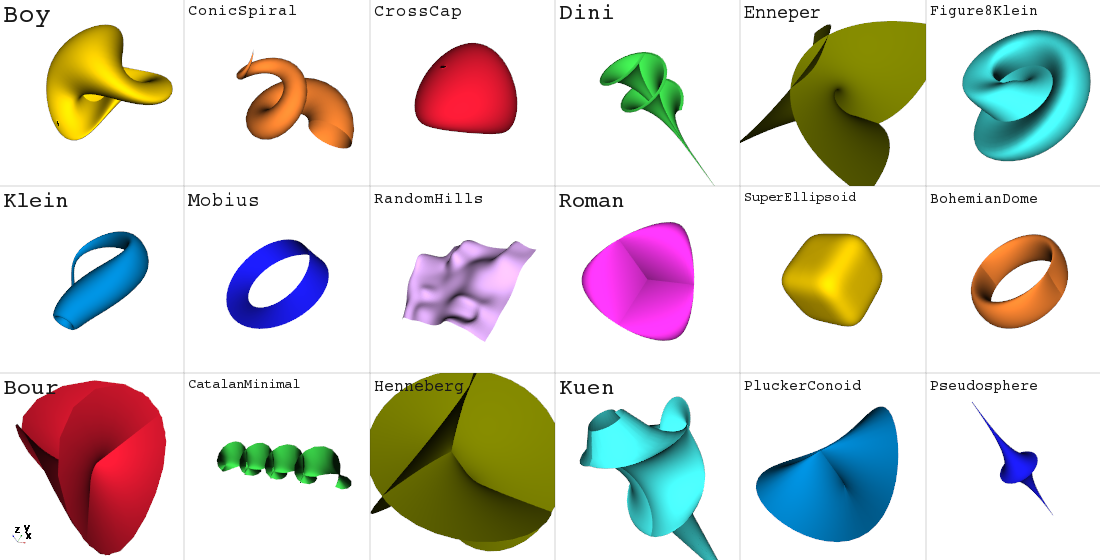## Plane¶

class vedo.shapes.Plane(pos=0, 0, 0, normal=0, 0, 1, sx=1, sy=None, c='g', alpha=1)[source]

Bases: vedo.mesh.Mesh

Draw a plane of size sx and sy oriented perpendicular to vector normal and so that it passes through point pos.## Polygon¶

class vedo.shapes.Polygon(pos=0, 0, 0, nsides=6, r=1, c='coral', alpha=1)[source]

Bases: vedo.mesh.Mesh

Build a polygon in the xy plane of nsides of radius r.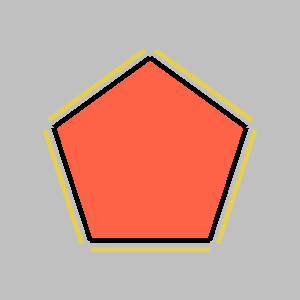## Pyramid¶

class vedo.shapes.Pyramid(pos=0, 0, 0, s=1, height=1, axis=0, 0, 1, c='dg', alpha=1)[source]

Build a pyramid of specified base size s and height, centered at pos.

## Rectangle¶

vedo.shapes.Rectangle(p1=0, 0, p2=2, 1, c='g', alpha=1)[source]

Build a rectangle in the xy plane identified by two corner points.

## Ribbon¶

class vedo.shapes.Ribbon(line1, line2=None, mode=0, closed=False, width=None, c='m', alpha=1, res=200, 5)[source]

Bases: vedo.mesh.Mesh

Connect two lines to generate the surface inbetween. Set the mode by which to create the ruled surface.

It also works with a single line in input. In this case the ribbon is formed by following the local plane of the line in space.

Parameters
• mode (int) – If mode=0, resample evenly the input lines (based on length) and generates triangle strips. If mode=1, use the existing points and walks around the polyline using existing points.

• closed (bool) – if True, join the last point with the first to form a closed surface

• res (list) – ribbon resolutions along the line and perpendicularly to it.

## Sphere¶

class vedo.shapes.Sphere(pos=0, 0, 0, r=1, c='r', alpha=1, res=24, quads=False)[source]

Bases: vedo.mesh.Mesh

Build a sphere at position pos of radius r.

Parameters
• float (r) – sphere radius

• res (int) – resolution in phi, resolution in theta is 2*res

## Spheres¶

class vedo.shapes.Spheres(centers, r=1, c='r', alpha=1, res=8)[source]

Bases: vedo.mesh.Mesh

Build a (possibly large) set of spheres at centers of radius r.

Either c or r can be a list of RGB colors or radii.

## Spline¶

class vedo.shapes.Spline(points, smooth=0.5, degree=2, closed=False, s=2, res=None)[source]

Return an Mesh for a spline which does not necessarly passing exactly through all the input points. Needs to import scypi.

Parameters
• smooth (float) –

smoothing factor.

• 0 = interpolate points exactly.

• 1 = average point positions.

• degree (int) – degree of the spline (1<degree<5)

• res (int) – number of points on the spline

## Spring¶

class vedo.shapes.Spring(startPoint=0, 0, 0, endPoint=1, 0, 0, coils=20, r=0.1, r2=None, thickness=None, c='grey', alpha=1)[source]

Bases: vedo.mesh.Mesh

Build a spring of specified nr of coils between startPoint and endPoint.

Parameters
• coils (int) – number of coils

• r (float) – radius at start point

• r2 (float) – radius at end point

• thickness (float) – thickness of the coil section

## Star¶

class vedo.shapes.Star(pos=0, 0, 0, n=5, r1=0.7, r2=1.0, line=False, c='m', alpha=1)[source]

Bases: vedo.mesh.Mesh

Build a 2D star shape of n cusps of inner radius r1 and outer radius r2.

Parameters

line (bool) – only build the outer line (no internal surface meshing).

## Star3D¶

class vedo.shapes.Star3D(pos=0, 0, 0, r=1.0, thickness=0.1, c='b', alpha=1)[source]

Bases: vedo.mesh.Mesh

Build a 3D star shape of 5 cusps, mainly useful as a 3D marker.

## Tensors¶

class vedo.shapes.Tensors(domain, source='ellipsoid', useEigenValues=True, isSymmetric=True, threeAxes=False, scale=1, maxScale=None, length=None, c=None, alpha=1)[source]

Bases: vedo.mesh.Mesh

Geometric representation of tensors defined on a domain or set of points. Tensors can be scaled and/or rotated according to the source at eache input point. Scaling and rotation is controlled by the eigenvalues/eigenvectors of the symmetrical part of the tensor as follows:

For each tensor, the eigenvalues (and associated eigenvectors) are sorted to determine the major, medium, and minor eigenvalues/eigenvectors. The eigenvalue decomposition only makes sense for symmetric tensors, hence the need to only consider the symmetric part of the tensor, which is 1/2*(T+T.transposed()).

Parameters
• source (str) – preset type of source shape [‘ellipsoid’, ‘cylinder’, ‘cube’ or any specified Mesh]

• useEigenValues (bool) – color source glyph using the eigenvalues or by scalars.

• threeAxes (bool) –

if False scale the source in the x-direction, the medium in the y-direction, and the minor in the z-direction. Then, the source is rotated so that the glyph’s local x-axis lies along the major eigenvector, y-axis along the medium eigenvector, and z-axis along the minor.

If True three sources are produced, each of them oriented along an eigenvector and scaled according to the corresponding eigenvector.

• isSymmetric (bool) – If True each source glyph is mirrored (2 or 6 glyphs will be produced). The x-axis of the source glyph will correspond to the eigenvector on output.

• length (float) – distance from the origin to the tip of the source glyph along the x-axis

• scale (float) – scaling factor of the source glyph.

• maxScale (float) – clamp scaling at this factor.

## Text¶

class vedo.shapes.Text(txt, pos=0, 0, 0, s=1, font='', hspacing=1.15, vspacing=2.15, depth=0, italic=False, justify='bottom-left', c=None, alpha=1)[source]

Bases: vedo.mesh.Mesh

Generate a 3D polygonal Mesh representing a text string.

Can render strings like 3.7 10^9 or H_2 O with subscripts and superscripts. Most Latex symbols are also supported (e.g. mu_lambda). Symbols ~ ^ _ are reserved modifiers:

use ~ to add a short space, 1/4 of the default empty space, use ^ and _ to start up/sub scripting, a space terminates their effect.

Parameters
• pos (list) – position coordinates in 3D space

• s (float) – size of text.

• depth (float) – text thickness.

• italic (bool,float) – italic font type (can be a signed float too).

• justify (str) – text justification as centering of the bounding box (bottom-left, bottom-right, top-left, top-right, centered).

• font (str) – available 3D-polygonized fonts are Bongas, Calco, Comae, Kanopus, Glasgo, LionelOfParis, Inversionz, LogoType, Normografo, Quikhand, SmartCouric, Theemim, VictorMono, VTK. Default is Normografo, which can be changed using settings.defaultFont

• hspacing (float) – horizontal spacing of the font.

• vspacing (float) – vertical spacing of the font for multiple lines text.

## Text2D¶

vedo.shapes.Text2D(txt, pos=3, s=1, c=None, alpha=0.15, bg=None, font='', justify='bottom-left', bold=False, italic=False)[source]

Returns a vtkActor2D representing 2D text.

Parameters
• pos (list, int) –

text is placed in one of the 8 positions:

1, bottom-left 2, bottom-right 3, top-left 4, top-right 5, bottom-middle 6, middle-right 7, middle-left 8, top-middle

If a pair (x,y) is passed as input the 2D text is place at that position in the coordinate system of the 2D screen (with the origin sitting at the bottom left).

• s (float) – size of text.

• bg – background color

• alpha (float) – background opacity

• justify (str) – text justification

• font (str) –

available fonts are

• Biysk

• Bongas

• Calco

• Comae

• Glasgo

• Inversionz

• Kanopus

• LionelOfParis

• LogoType

• Normografo

• Quikhand

• SmartCouric

• Theemim

• VictorMono

A path to otf or ttf font-file can also be supplied as input.

## Torus¶

class vedo.shapes.Torus(pos=0, 0, 0, r=1, thickness=0.2, c='khaki', alpha=1, res=30)[source]

Bases: vedo.mesh.Mesh

Build a torus of specified outer radius r internal radius thickness, centered at pos.

## Tube¶

class vedo.shapes.Tube(points, r=1, cap=True, c=None, alpha=1, res=12)[source]

Bases: vedo.mesh.Mesh

Build a tube along the line defined by a set of points.

Parameters